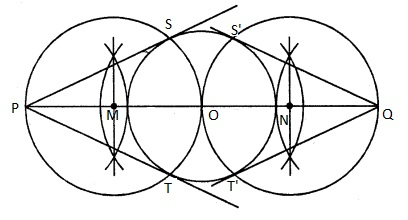# Draw a circle of radius 3 cm. Take two points P and Q on one of its extended diameter each at a distance of 7 cm from its centre. Draw tangents to the circle from these two points P and Q.

Given:

A circle of radius 3 cm. Take two points P and Q on one of its extended diameter each at a distance of 7 cm from its centre.

To do:

We have to draw a circle of radius 3 cm and draw tangents to the circle from the points P and Q.

Solution:Steps of construction:

(i) Draw a circle with centre $O$ and radius $3\ cm$.

(ii) Draw a diameter and produce it to both sides.

(iii) Take two points $P$ and $Q$ on this diameter with a distance of $7\ cm$ each from the centre $O$.

(iv) Bisect $PO$ at $M$ and $QO$ at $N$.

(v) With centres $M$ and $N$, draw circle on $PO$ and $QO$ as diameter which intersect the given circle at $S, T$ and $S’, T’$ respectively.

(vi) Join $PS, PT, QS’$ and $QT’$.

$PS, PT, QS’$ and $QT’$ are the required tangents to the given circle.

Updated on: 10-Oct-2022

45 Views